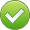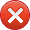# Percentage Calculator online

### Percentage Calculator

What is  % of ?
0% from 0 = 0is what % of ?
0 from 0 = 0%Add 0% to 0 = 0Deduct % to
Deduct 0% to 0 = 0What is the percentage increase/decrease
from to ?
Increase 0 to 0 = 0%Percentage calculator enables making various calculations involving percentage. In terms of functionality, the percentage calculator consists of four different calculators and makes it possible to calculate:
- what number is X percent from Y number;
- how many percent is X number from Y number;
- add X percent to Y number;
- deduct X percent from Y number.

Calculation results may be reduced to the decimal digits you need. Below you can find detailed instruction and examples of the percentage calculator operation.

## Instruction on percentage calculator operation

In a pop-down menu choose the mode of percentage calculation you need.
Enter X number and Y number in the corresponding cells.
Choose the calculation accuracy by defining the necessary number of decimal digits in the dedicated pop-down menu.
Percentage calculator makes calculations while you are entering numbers in the cells and the result is shown immediately.
You can copy the result by clicking on the sum total.
The calculations made may be saved, deleted, and adjusted in percentage calculator’s memory.
The previous calculation will be saved.
If necessary, it is possible to delete the values entered by pressing "Clear" button.

### Examples of percentage calculator operation

Example 1
Which number corresponds to 58 % from 240.2591? Reduce to 4 decimal digits.
Result - 139.3503.

Example 2
How many percent is 258 from 457.2578. Reduce to 6 decimal digits.
Result - 56.423313 %.

Example 3
Add 27 % to 534. Reduce to 2 decimal digits.
Result- 678.18.

Example 4
Deduct 18 % from 3587.4578. Reduce to 4 decimal digits.
Result - 2941.7154.

### How to calculate percentage without percentage calculator

What number corresponds to 18 % from 450?
1. Deduce the coefficient: 450 / 100 = 4.5
2. Get the final value: 4.5 х 18 = 81
Result: 81.

How many percent is 45 from 500?
1. Deduce the coefficient: 500 / 45 = 11.1111
2. Get the final value: 100 % / 11.1111 = 9
Result: 9

1. Deduce the coefficient: 572 / 100 = 5.72
2. Get the number equal to 12 %: 12 % x 5.72 = 68.64м
3. Get the final value: 572 + 68.64 = 640.64
Result: 640.64

Deduct 45 % from 2870
1. Deduce the coefficient: 2870 / 100 = 28.7
2. Get the number equal to 45 %: 45 x 28.7 = 1291.5
3. Get the final value: 2870 - 1291.5 = 1578.5
Result: 1578.5

### Genesis of percentage calculations

The origins of percentage calculations go back to ancient Babylon. Translated from Latin, percentage means "per hundred" or "from hundred". By means of cuneiform tablets made by Babylonians that survived until the present day, it was easy to find out the sum of yield.

The second hypothesis of percentage origins leads us to India. Indian accountants calculated percentage using the rule of three based on proportion.

The third, and, perhaps, the most reliable story, arrived to us from ancient Rome. Percentage emergence was provoked when the senate established the maximum allowed sum of money to be collected from debtor by creditor in excess of the debt amount.